﻿ 相位锁定光电振荡器的仿真分析与优化 Simulation Analysis and Optimization of Phase Locked Photoelectric Oscillator

Optoelectronics
Vol.08 No.03(2018), Article ID:26663,9 pages
10.12677/OE.2018.83013

Simulation Analysis and Optimization of Phase Locked Photoelectric Oscillator

Chenyi Cai

Nanjing University of Science and Technology, Nanjing Jiangsu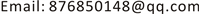Received: Aug. 6th, 2018; accepted: Aug. 23rd, 2018; published: Aug. 30th, 2018ABSTRACT

The phase noise transfer function of the photoelectric oscillator based on injection locking technique and phase-locked loop technique are derived respectively in this paper. The simulation compares the phase noise performance of free running OEO and locked OEO. Due to the high sensitivity of the fiber to temperature, there is a situation in which the lock is easy to lose. This paper proposes an improved injection-locked OEO scheme to improve system frequency stability. Considering the importance of the RF filter in the loop system, an X-band narrowband filter with a bandwidth of 20 MHz is used to improve the overall performance of the phase-locked loop.

Keywords:Optoelectronic Oscillator, Phase Noise, Injection Locked, Phase-Locked Loop, Narrowband Filter1. 引言

2. 注入锁定

2.1. 自注入锁定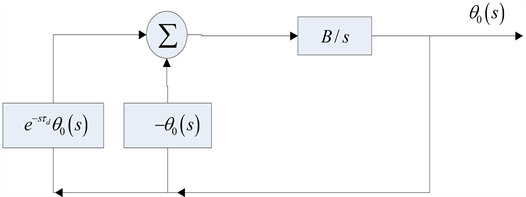Figure 1. Self-injection locked oscillator block diagram

${\theta }_{01}\left(s\right)=-\frac{B}{s}{\theta }_{01}\left(s\right)+{\text{e}}^{-s{\tau }_{d}}{\theta }_{01}\left(s\right)+\frac{B}{s}{n}_{1}\left(s\right)$ (1)

${\theta }_{o2}\left(s\right)=-\frac{B}{s}{\theta }_{o2}\left(s\right)+{\text{e}}^{-s{\tau }_{d}}\frac{B}{s}{\theta }_{o2}\left(s\right)+{n}_{2}\left(s\right)$ (2)

${\theta }_{0}$ 的功率谱密度可以表示为：

${S}_{sil}\left({\omega }_{m}\right)={|{H}_{a}\left(s\right)|}^{2}{S}_{n1}\left({\omega }_{m}\right)+{|{H}_{b}\left(s\right)|}^{2}{S}_{n2}\left({\omega }_{m}\right)$ (3)

${H}_{a}\left(s\right)=\frac{B}{s+B\left(1-{\text{e}}^{-s{\tau }_{d}}\right)}$ (4)

${H}_{b}\left(s\right)=\frac{s}{s+B\left(1-{\text{e}}^{-s{\tau }_{d}}\right)}$ (5)

${S}_{n1}\left({f}_{m}\right)$ 为频偏 ${f}_{m}$ 处的剩余噪声：

${S}_{n1}\left({f}_{m}\right)=\frac{kT{B}^{\prime }F}{2{P}_{s}}\left(\frac{{f}_{c}}{{f}_{m}}+1\right)$ (6)

${S}_{n2}\left({f}_{m}\right)$ 为振荡器在频偏 ${f}_{m}$ 处的相位噪声  ：

${S}_{n2}\left({f}_{m}\right)=\frac{\delta }{1+{|G\left({V}_{0}\right)|}^{2}-2|G\left({V}_{0}\right)|\mathrm{cos}\left(2\text{π}{f}_{m}\tau \right)}+\frac{\alpha \cdot \delta /f}{1+{|G\left({V}_{0}\right)|}^{2}-2|G\left({V}_{0}\right)|\mathrm{cos}\left(2\text{π}{f}_{m}\tau \right)}$ (7)

2.2. 双自注入锁定

${S}_{sil}\left({\omega }_{m}\right)={|{H}_{a}\left(s\right)|}^{2}{S}_{n1}\left({\omega }_{m}\right)+{|{H}_{b}\left(s\right)|}^{2}{S}_{n2}\left({\omega }_{m}\right)$ (8)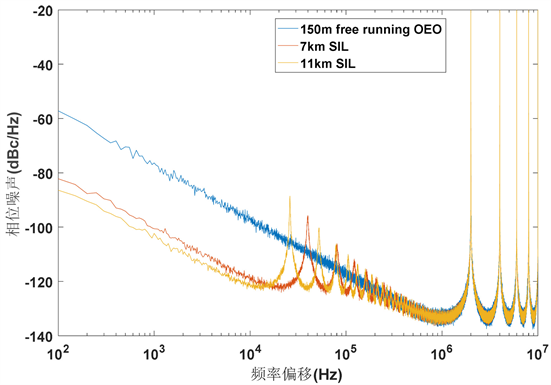Figure 2. Phase noise simulation of 150 m free running OEO, 7 km SIL OEO and 11 km SIL OEO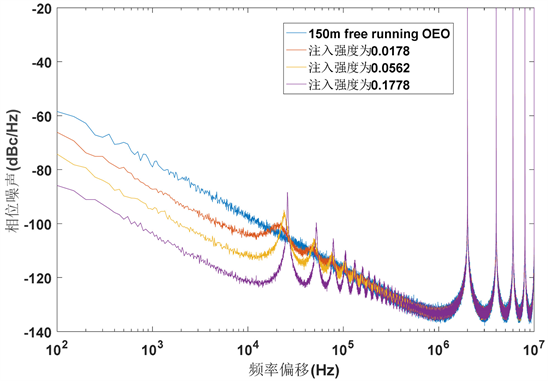Figure 3. Phase noise simulation of 150 m OEO and 11 km SIL OEO at different injection intensities

${H}_{a}\left(s\right)=\frac{2B}{2s+B\left(1-{\text{e}}^{-s{\tau }_{d1}}\right)+B\left(1-{\text{e}}^{-s{\tau }_{d2}}\right)}$ (9)

${H}_{b}\left(s\right)=\frac{2s}{2s+B\left(1-{\text{e}}^{-s{\tau }_{d1}}\right)+B\left(1-{\text{e}}^{-s{\tau }_{d2}}\right)}$ (10)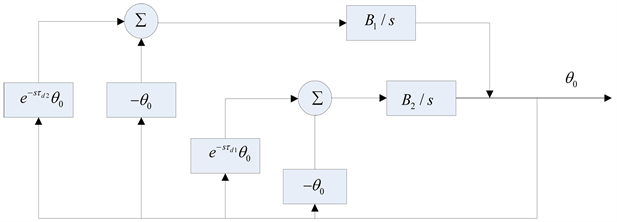Figure 4. Dual self-injection locked oscillator system block diagram

150 m环长OEO采用7 km和11 km光纤延迟线进行双自注入锁定，并单独使用7 km和11 km的光纤延迟线进行自注入锁定，通过matlab仿真对相噪图进行比较。可以看出在双自注入锁定OEO中，杂散模式的抑制较好。双自注入锁定OEO的最好相噪水平决定于其中最长的延迟线的长度以及两个自注入环路的功率分配。本文中两路自注入锁定的功率是相等的，所以双自注入锁定OEO的相噪水平是两段光纤分别进行注入锁定的平均(图5)。

3. 锁相环光电振荡器

$H\left(s\right)=\frac{{K}_{pd}{K}_{OEO}F\left(s\right)}{s}$ (11)

${H}_{OEO}\left(s\right)=\frac{1}{1+H\left(s\right)}$ (12)

${H}_{REF}\left(s\right)=\frac{H\left(s\right)}{1+H\left(s\right)}$ (13)

${H}_{PD}\left(s\right)={H}_{LF}\left(s\right)=\frac{H\left(s\right)}{1+H\left(s\right)}\cdot \frac{1}{{K}_{pd}}$ (14)

$\Delta {\theta }_{out}=\Delta {\theta }_{OEO}{H}_{OEO}\left(s\right)+\Delta {\theta }_{REF}{H}_{REF}\left(s\right)+\Delta {\theta }_{PD}{H}_{PD}\left(s\right)+\Delta {\theta }_{LF}{H}_{LF}\left(s\right)$ (15)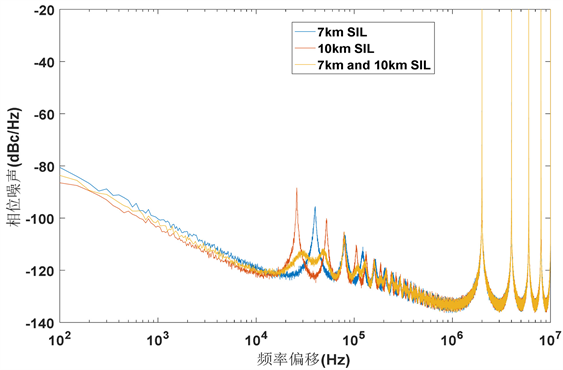Figure 5. Phase noise comparison between dual self-injection locking and single self-injec- tion-locked OEO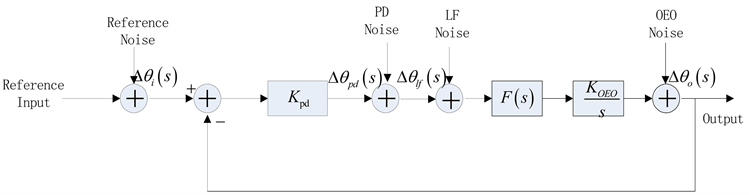Figure 6. S-domain noise model of phase-locked loop OEO

4. 失锁和改进的方法

OEO锁定后的输出频率与参考频率相同，则OEO的频率稳定性由外部参考源的稳定性决定。外部源通常由高稳晶振倍频产生。锁定后稳定性远远优于自由运行的OEO，则希望OEO的锁定带宽能足够的大。但是带宽一味增大会使OEO得相噪性能恶化，所以一般情况下锁定带宽不超过5 kHz，在温度稳定的实验环境下，OEO很容易失锁，一般只能维持几分钟的锁定时间。为了解决该问题本文搭建了锁定状态检测反馈回路，保证OEO频率稳定在锁定频率内。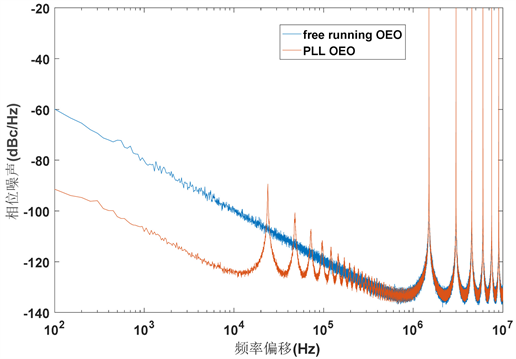Figure 7. Comparison of phase noise between OEO and phase-locked loop OEO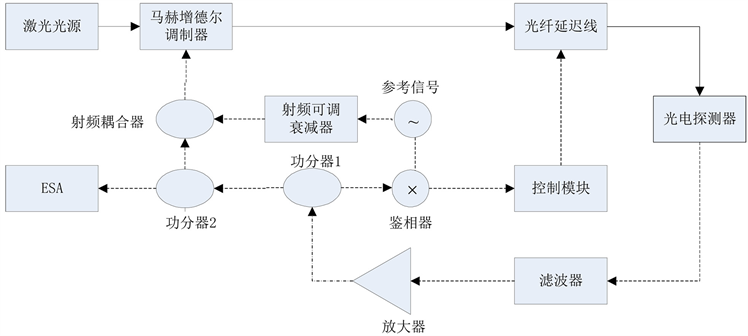Figure 8. Improved injection-locked photoelectric oscillator structure

5. 窄带带通滤波器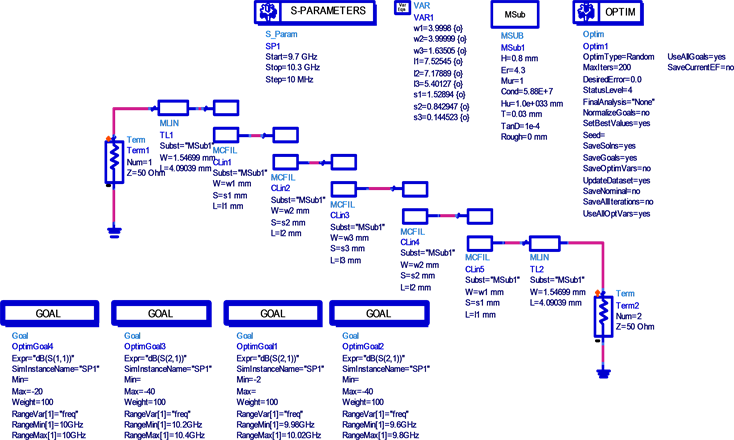Figure 9. Bandpass filter circuit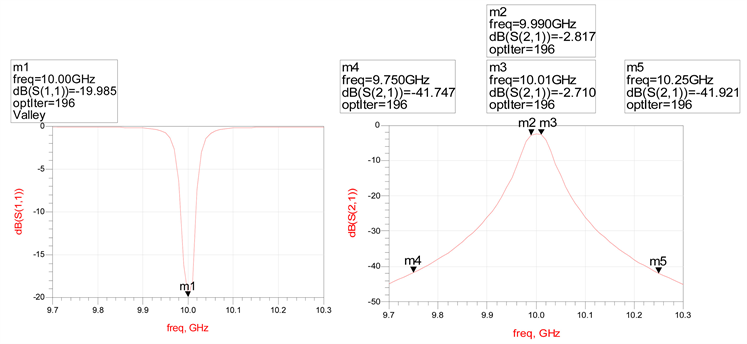Figure 10. Bandpass filter simulation circuit diagram

6. 结论

Simulation Analysis and Optimization of Phase Locked Photoelectric Oscillator[J]. 光电子, 2018, 08(03): 89-97. https://doi.org/10.12677/OE.2018.83013

1. 1. Neyer, A. and Voges, E. (1982) Dynamics of Electroptic Bistable Devices with Delayed Feedback. IEEE Journal of Quantum Elec-tronics, 18, 2009-2015. https://doi.org/10.1109/JQE.1982.1071487

2. 2. Yao, X.S. and Maleki, L. (2000) Multiloop Optoelec-tronic Oscillator. IEEE Journal of Quantum Electronics, 36, 79-84. https://doi.org/10.1109/3.817641

3. 3. Cai, S., Pan, S., Zhu, D., et al. (2012) Coupled Frequency-Doubling Optoelectronic Os-cillator Based on Polarization Modulation and Polarization Multiplexing. Optics Communications, 285, 1140-1143. https://doi.org/10.1016/j.optcom.2011.11.039

4. 4. Qu, S., Jin, T., Chi, H., et al. (2015) An Optoelectronic Oscillator Using an FBG and an FBG-Based Fabry-Perot Filter. Optics Communications, 342, 141-143. https://doi.org/10.1016/j.optcom.2014.12.063

5. 5. Kim, T.H., Lee, S., Lee, C.H., et al. (2016) Dual Loop Optoelectronic Os-cillator with Acousto-Optic Delay Line. Journal of the Optical Society of Korea, 20, 300-304. https://doi.org/10.3807/JOSK.2016.20.2.300

6. 6. Ohta, T. and Murakami, K. (1968) Reducing Negative-Resistance Oscillator Noise by Self Injection.

7. 7. 杨波. 新型光电振荡器及其在光载射频传输系统中的应用研究[D]: [博士学位论文]. 杭州: 浙江大学, 2013.